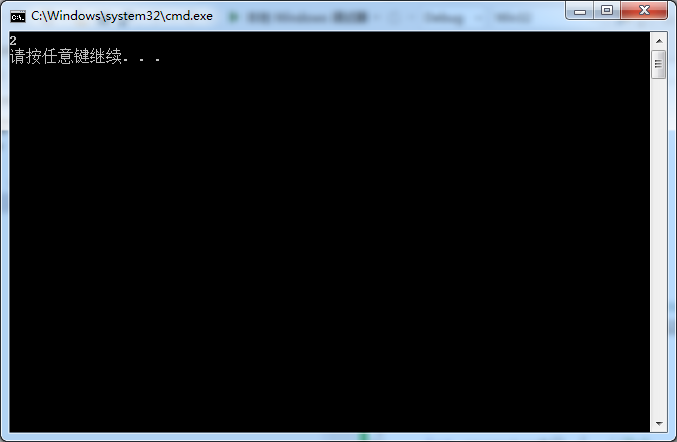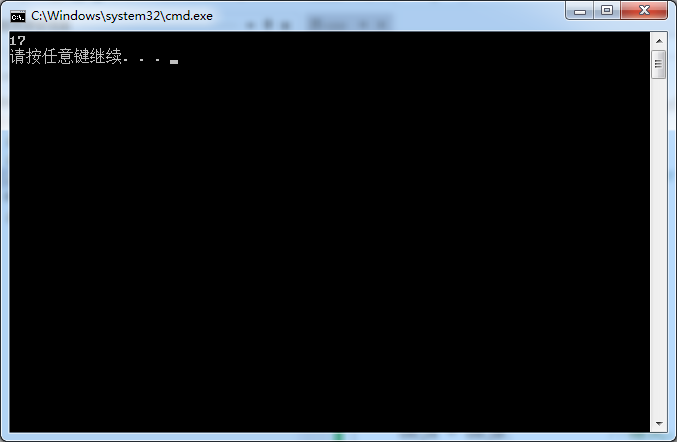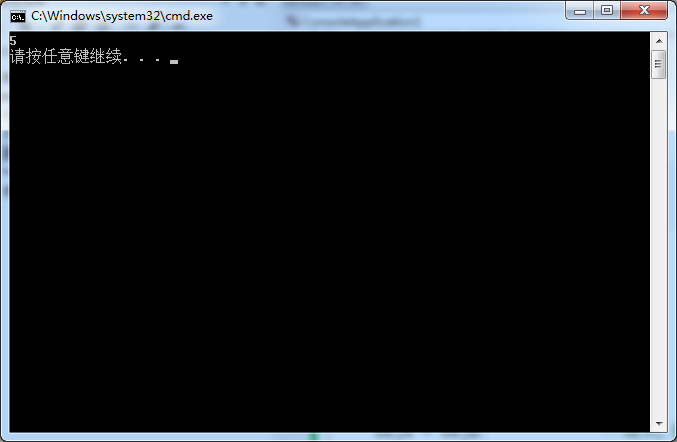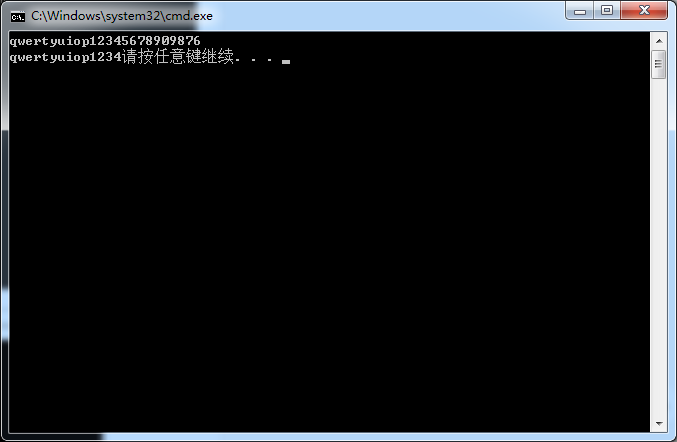## 多态及运算符重载

C++ 多态的定义及实现
1. 多态定义的构成条件

2.析构函数重写问题

3.抽象类

#include <ostream>
#include <iostream>
using namespace std;

class CCounter {
public:
CCounter() {
m_nValue = 0;
};
friend void operator++(CCounter &obj) {     //1th  由于友元函数不属于此类,故这里要加引用符号
if (obj.m_nValue < 65535)
{
obj.m_nValue++;
}
}
int operator*() {  //2th
return m_nValue;
}

private:
int m_nValue;
};

int main()
{
CCounter objA;
++objA;         //隐式，调用1th
operator++(objA);//显示调用1th,这里需要加objA,这里与1th处与成员函数运算符重载不同!!!
cout << *objA << endl;      //调用2th处函数
return 0;
}双目：

#include <iostream>
using namespace std;

class CCounter {
public:
CCounter(int nNum) {
m_nValue = nNum;
};
friend void operator+(CCounter &objA,CCounter objB) {       //1th  由于友元函数不属于此类,故这里要加引用符号
//这里第二个&可以不用加!

objA.m_nValue+=objB.m_nValue;

}
friend int operator*(CCounter obj) {  //2th
return obj.m_nValue;
}

private:
int m_nValue;
};

int main()
{
CCounter objA(5),objB(6);
objA+objB;          //隐式，调用1th
operator+(objA,objB);//显示调用1th,这里需要加objA,这里与1th处与成员函数运算符重载不同!!!
cout << *objA << endl;      //调用2th处函数
return 0;
}特殊符号:

#include <iostream>
using namespace std;

class CCounter {
public:
CCounter(int nNum) {
m_nValue = nNum;
}
CCounter(CCounter &obj) {
m_nValue = obj.m_nValue;
}

CCounter& operator=(CCounter &obj) {        //1th

m_nValue = obj.m_nValue;
return *this;

}
int operator *() {
return m_nValue;
}

private:
int m_nValue;
};

int main()
{
CCounter objA(15), objB(5);
objA = objB;            //隐式,调用1th
cout << *objA << endl;  //调用2处函数
return 0;
}特殊运算符重载：

#include <iostream>
using namespace std;

class CCounter {
public:
CCounter() {
memset(m_szStr, 0, sizeof(m_szStr));//0th
}

friend ostream& operator<<(ostream& output, CCounter &obj) {//1th
output << obj.m_szStr;
return output;

}

friend istream& operator>>(istream& input, CCounter &obj) {//2th
input.getline(obj.m_szStr, 15);//获取一行的数据(这里指字母或者数字的个数,包括'\0')
//输入的个数大于15,只是获取前面15个!!!
return input;

}

private:
char m_szStr;
};

int main()
{
CCounter obj;       //调用0th处的构造函数
cin >> obj;  //调用2th
cout << obj;  //调用1th
return 0;
}posted on 2019-10-27 16:29  lllyyynnn  阅读(503)  评论(0编辑  收藏  举报